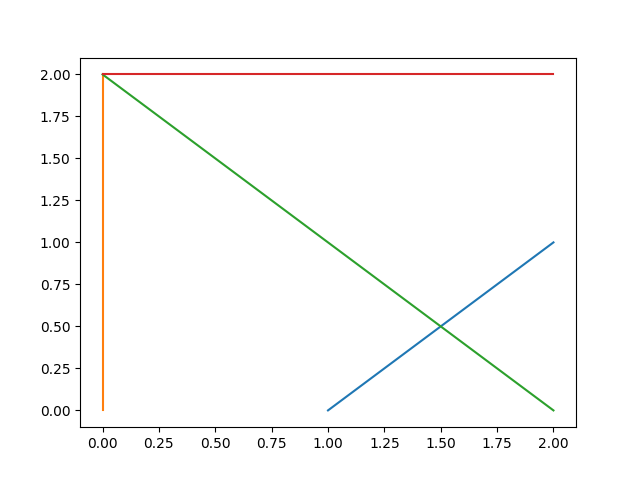At work there is a monthly puzzler.

“Design a robot that can pick up all 9 apples arranged on a 3 by 3 rectangular grid, and spaced 1m apart. The robot parts they have are faulty. The robot can only turn three times”

I think the intent of the puzzle is that the robot can drive in forward and reverse, but only actually turn 3 times. It’s not very hard to do by hand. I decided to take a crack at this one using Z3 for funzies. Z3 is an SMT solver. It is capable of solving a interesting wide variety of problems.

I interpret this as “find 4 lines that touch all points in the grid, such that each subsequent line intersects.”

It is fairly easy to directly translate this into a Z3 model.

from z3 import *
import matplotlib.pyplot as plt
import numpy as np
s = Solver()

linenum = 4

def linepoint(l,p): #line point product. Is zero if point is on line
return l*p + l*p + l

lines = [[Real(s + str(i)) for s in ('a','b','c')] for i in range(linenum)]

applepoints = [(x,y) for x in range(5) for y in range(3)]
turnpoints = [[Real(s + str(i)) for s in ('x','y')] for i in range(linenum-1)]

for pt in applepoints:  #Every point needs to be touched by one line

for l in lines: #Exclude invalid lines (all zeros)
s.add(Or([a != 0 for a in l]))

for i in range(linenum-1): #Consecutive lines intersect (aren't parallel). There are other ways of doing this

def convert(x):
if is_int_value(x):
return x.as_long()
elif is_rational_value(x):
return x.numerator_as_long()/x.denominator_as_long()
elif is_algebraic_value(x):
return convert(x.approx(7))

print(s.check())

m = s.model()
#print("x = %s" % m[x])

#print "traversing model..."
for d in m.decls():
print("%s = %s" % (d.name(), m[d]))

#nplines = np.array([[m[a].numerator_as_long()/m[a].denominator_as_long() for a in l] for l in lines])
nplines = np.array([[convert(m[a]) for a in l] for l in lines])

#xs = np.random.randn(2,3)
#xs = np.

print(nplines)
for l in nplines:
pts = []
for j in [-1,1]:
# We can use Z3 to draw a nice set of lines too
opt = Optimize()
x, y = Reals('x y')
opt.add([0 <=  x, x <= 2, 0 <= y, y <= 2])
opt.add(l * x + l*y + l == 0)
opt.minimize(j*0.453 * x + j*0.3234 * y) # random objective direction hopefully not perpendicular to a line
print(opt.check())
m = opt.model()
pts.append([convert(m[x]), convert(m[y])])
print(l)
pts = np.array(pts).T
plt.plot(pts[0,:], pts[1,:])
plt.show()Another interesting approach might be to note that the points are described by the set of equations $x*(x-1)*(x-2)=0$ and $y*(y-1)*(y-2)=0$ . I think we could then possibly use methods of nonlinear algebra (Groebner bases) to find the lines. Roughly an ideal containment question? Don’t have this one fully thought out yet. I think z3 might be doing something like this behind the scenes.